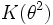# Galois extensions for dihedral group:D8

## Contents

View Galois extensions for particular groups | View other specific information about dihedral group:D8

This article discuss various examples of Galois extensions of fields for which the Galois group is dihedral group:D8.

## Fourth roots of a non-square

Suppose$K$ is a field of characteristic not equal to two, such that$-1$ is not a square in$K$. Suppose$b$ is an element of$K$ such that neither$b$ nor$-b$ is a square in$K$. Then, the extension:$K(\theta,i)$

where$\theta$ is a fourth root of$b$ and$i$ is a squareroot of$-1$, is a Galois extension whose automorphism group is the dihedral group of order eight.

A concrete example is$\mathbb{Q}(2^{1/4},i)$.

### Description of the automorphisms

The dihedral group is given by the presentation:$\langle a,x \mid a^4 = x^2 = 1, xax = a^{-1} \rangle$.

Here,$a$ acts by fixing$i$ and sending$\theta$ to$i\theta$, while$x$ fixes$\theta$ and sends$i$ to$-i$.

In fact, the dihedral group acts on the set$\{ \theta, i\theta, -\theta, -i\theta \}$ precisely the way it acts on the vertices of a square. In the concrete case of$\mathbb{Q}(2^{1/4},i)$, these elements, when plotted in$\mathbb{C}$ do form the vertices of a square, so the Galois automorphisms correspond to the usual rotations and reflections.

### Galois correspondence for subgroups

For more on the subgroup structure, refer subgroup structure of dihedral group:D8.

1. The trivial subgroup corresponds to the whole field$K(\theta,i)$.
2. The center corresponds to the subfield$K(\theta^2,i)$.
3. The two-element subgroup generated by$x$ corresponds to the subfield$K(\theta)$. The two-element subgroup generated by$a^2x$ corresponds to the subfield$K(i\theta)$. The other two-element subgroups correspond to the subfields$K(\theta(1+i)/2)$ and$K(\theta(1-i)/2)$.
4. The four-element subgroup generated by$a^2$ and$x$ corresponds to$K(\theta^2)$.
5. The four-element subgroup generated by$a^2$ and$ax$ corresponds to$K(i\theta^2)$.
6. The cyclic four-element subgroup generated by$a$ corresponds to$K(i)$.
7. The whole group corresponds to$K$.